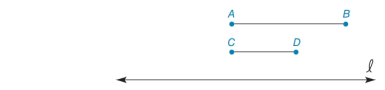Chapter 1.3, Problem 30E### Elementary Geometry for College St...

6th Edition
Daniel C. Alexander + 1 other
ISBN: 9781285195698

#### Solutions

Chapter
Section### Elementary Geometry for College St...

6th Edition
Daniel C. Alexander + 1 other
ISBN: 9781285195698
Textbook Problem
1 views

# In Exercises 29 to 32, use only a compass and a straightedge to complete each construction.Exercises 29, 30 Given: A B ¯   and   C D ¯ ( A B > C D ) Construct: E F ¯ on line l so that E F = A B − C D

To determine

To find:

The complete construction of EF¯ on line 𝓁 so that EF=ABCD.

Explanation

Given:

The given figure is,

AB¯andCD¯(AB>CD)

Approach:

The steps are given below for the complete construction.

a) Draw a straight line and label a point E.

b) Use compass and open it to the width of AB¯

### Still sussing out bartleby?

Check out a sample textbook solution.

See a sample solution

#### The Solution to Your Study Problems

Bartleby provides explanations to thousands of textbook problems written by our experts, many with advanced degrees!

Get Started

#### In Exercises 2340, find the indicated limit. 24. limx23

Applied Calculus for the Managerial, Life, and Social Sciences: A Brief Approach

#### The area of the region bounded by , , and r = sec θ is:

Study Guide for Stewart's Multivariable Calculus, 8th

#### In Exercises 110, evaluate the expression. C(52,7)

Finite Mathematics for the Managerial, Life, and Social Sciences